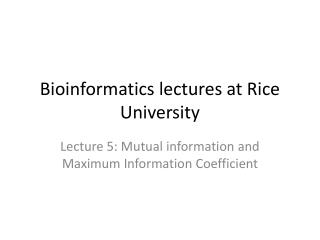DownloadDownload PresentationBioinformatics lectures at Rice University

# Bioinformatics lectures at Rice University

Télécharger la présentation## Bioinformatics lectures at Rice University

- - - - - - - - - - - - - - - - - - - - - - - - - - - E N D - - - - - - - - - - - - - - - - - - - - - - - - - - -
##### Presentation Transcript

1. Bioinformatics lectures at Rice University Lecture 5: Mutual information and Maximum Information Coefficient

2. -- from Science 16 December 2011

3. How to compute MIC

4. Equitability and Generality

5. Example

6. Example: Yeast Gene expression cell cycle data MIC can find new relationships

7. Using the mode value for calibration instead of the mean or the median We found the mode value to be useful for calibrating copy number data. In normal cells, the DNA copy number is 2 in most of the regions in the genome. A common method for calibration is to set the average value or the median value to be 2. However, when a substantial fraction is altered in cancer, the method no longer works. With denoised data, the distribution of copy number data have multiple peaks. The dominant peak is the optimal choice for calibrating the data. In addition, because normal regions should not have allelic imbalance, we can further narrow down the peaks. median mode mean

8. Example 2: T-test vs. regularized t-test T-test is often used to test the significance of difference between two population means. s2 is the unbiased estimator of the variance of the two samples, ni= number of elements in group i, i=1 or 2. When the sample size is small, variance estimate is unstable, which renders t-test significance unstable. In the analysis of microarray gene expression data, researchers use a large number of t-tests to identify significant differences between two groups of samples. A Bayesian approach, regularized t-test, can be used to stabilize the variance estimate, assuming that gene with similar values have similar variability. The new approach can reduce false positives.

9. Example 2: T-test vs. regularized t-test T-test is often used to test the significance of difference between two population means. s2 is the unbiased estimator of the variance of the two samples, ni= number of elements in group i, i=1 or 2. When the sample size is small, variance estimate is unstable, which renders t-test significance unstable. In the analysis of microarray gene expression data, researchers use a large number of t-tests to identify significant differences between two groups of samples. A Bayesian approach, regularized t-test, can be used to stabilize the variance estimate, assuming that gene with similar values have similar variability. The new approach can reduce false positives.

10. Use of sequence information to model genomics data • The number of features per biological specimen is often very large 105~109. The number of specimens is usually much small, less than a few hundreds. • The raw data are often uncalibrated, i.e., they do not come with meaningful units. • Biases can occur due to degradation of specimen, distorted amplification and biased sampling. • The biases to a great extent depend on the DNA sequence of the specific locus measured in microarrays or NGS reads, which can be quantified through modeling.

11. Positional Dependant Nearest-Neighbor (PDNN) model of molecular interactions Model of free energy is expressed as a weighted sum base-pair stacking energies, where the weights depend on the nucleotide position. PDNN model DNA double helix Model predicted values fit obs

12. Discovery of molecular insights through modeling the data Cross hybridization tends to cling to the tips of the probes on microarray surface Amplification bias on SNP arrays depends on the length of target molecule exercised by the restriction enzyme The attachment of a probe on the microarray surface affects the binding affinity of the probe

13. Correlated mutations

14. Direct Information

15. HOME WORK 2: The home work is set to test use MIC algorithm. Please see online material for details. http://odin.mdacc.tmc.edu/llzhang/RiceCourse/HMWK2